## Monday, July 25, 2016

### Update to `plotTree.boxplot` for `formula` method

In the documentation to the new function `plotTree.boxplot` I noted:

“\code{x} can also be supplied as a formula, though in that case the factor levels need to be provided in the cladewise order of the tips in \code{tree}.”

What I didn't note is that if this is not done, then the result will be incorrect without any error being reported. This is because the `formula` provided by the user is passed directly to the function `boxplot` without any feedback to the tree plotter.

What I realized is that one thing that I could do was first run `boxplot.formula` with `plot=FALSE` which (invisibly) returns a list containing the order of the plotted boxes. Then I could pass that argument to `plotTree` using `tips`. Finally, I could re-run `boxplot` with `plot=TRUE`. If the order of the factor in `formula` is a valid cladewise order of the tips of the tree then the tree & boxplot will plot fine. If it is not, say if we randomize the levels of our factor, then the edges of the tree will cross when plotting.

Here is what I mean.

Here's our tree & data applied using the basic method:

``````library(phytools)
tree
``````
``````##
## Phylogenetic tree with 26 tips and 25 internal nodes.
##
## Tip labels:
##  Z, Y, X, W, V, U, ...
##
## Rooted; includes branch lengths.
``````
``````x[1:30] ## first 30 elements of x
``````
``````##            Z            Z            Z            Z            Z
##  1.481718482  1.999288805  1.244922289  1.465959575  1.439767680
##            Y            Y            Y            Y            Y
##  1.014757614  1.937999703  0.728102930  0.690196494 -0.081170095
##            X            X            X            X            X
##  1.506780336  1.680023687  1.337899661  0.300120161  0.706118301
##            W            W            W            W            W
## -0.615363928  1.062724934 -0.556182889 -1.070714742 -1.271925923
##            V            V            V            V            V
## -1.228228110 -0.957836899 -0.617736401  0.300541684 -0.161967102
##            U            U            U            U            U
##  1.722133482  1.169447096  0.624685958  2.154831186  0.009577268
``````
``````plotTree.boxplot(tree,x) ## basic method
``````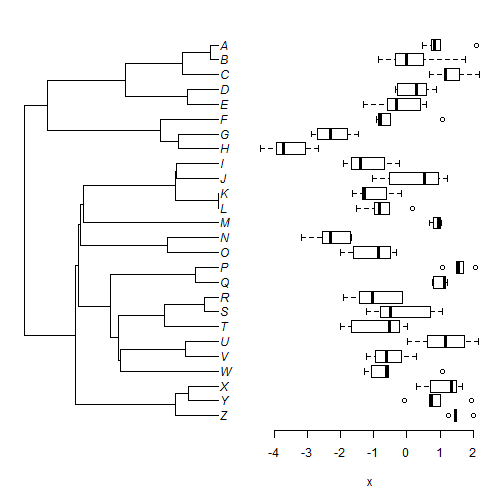Now let's try the formula method in which our factor is in the order of our tree's tip labels:

``````spp<-factor(names(x),levels=reorder(tree)\$tip.label)
plotTree.boxplot(tree,x~spp)
``````Next, we can flip the order of our factor levels. This is still a valid cladewise order so this should work fine:

``````spp<-factor(names(x),levels=tree\$tip.label[Ntip(tree):1])
spp
``````
``````##    Z Z Z Z Z Y Y Y Y Y X X X X X W W W W W V V V V V U U U U U T T T T T
##   S S S S S R R R R R Q Q Q Q Q P P P P P O O O O O N N N N N M M M M M
##   L L L L L K K K K K J J J J J I I I I I H H H H H G G G G G F F F F F
##  E E E E E D D D D D C C C C C B B B B B A A A A A
## Levels: A B C D E F G H I J K L M N O P Q R S T U V W X Y Z
``````
``````plotTree.boxplot(tree,x~spp)
``````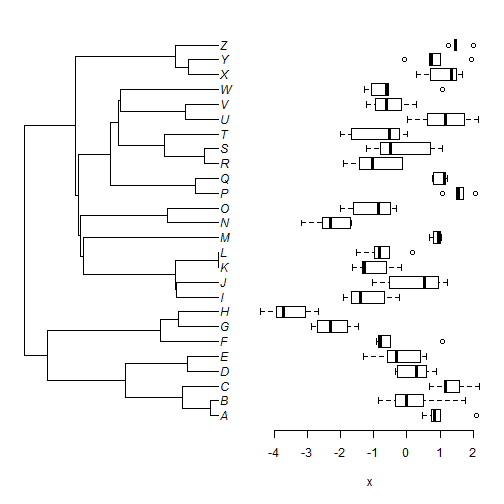``````spp<-factor(names(x),untangle(ladderize(tree),"read.tree")\$tip.label)
spp
``````
``````##    Z Z Z Z Z Y Y Y Y Y X X X X X W W W W W V V V V V U U U U U T T T T T
##   S S S S S R R R R R Q Q Q Q Q P P P P P O O O O O N N N N N M M M M M
##   L L L L L K K K K K J J J J J I I I I I H H H H H G G G G G F F F F F
##  E E E E E D D D D D C C C C C B B B B B A A A A A
## Levels: V U W S R T Q P L K J I M O N Y X Z B A C E D H G F
``````
``````plotTree.boxplot(tree,x~spp)
``````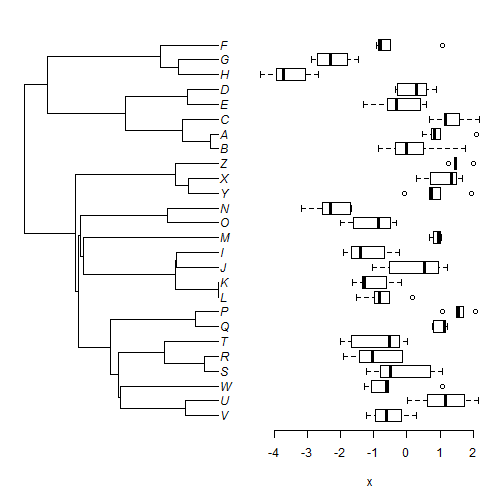(Note that we didn't ladderize our input tree for `plotTree.boxplot` - however the plotted tree will be ladderized.)

Finally, randomize them. This will not (generally speaking) result in a valid cladewise order:

``````spp<-factor(names(x),levels=sample(tree\$tip.label))
spp
``````
``````##    Z Z Z Z Z Y Y Y Y Y X X X X X W W W W W V V V V V U U U U U T T T T T
##   S S S S S R R R R R Q Q Q Q Q P P P P P O O O O O N N N N N M M M M M
##   L L L L L K K K K K J J J J J I I I I I H H H H H G G G G G F F F F F
##  E E E E E D D D D D C C C C C B B B B B A A A A A
## Levels: N L D C Q F J E T V A G P O B Y U K M S R H X W Z I
``````
``````plotTree.boxplot(tree,x~spp)
``````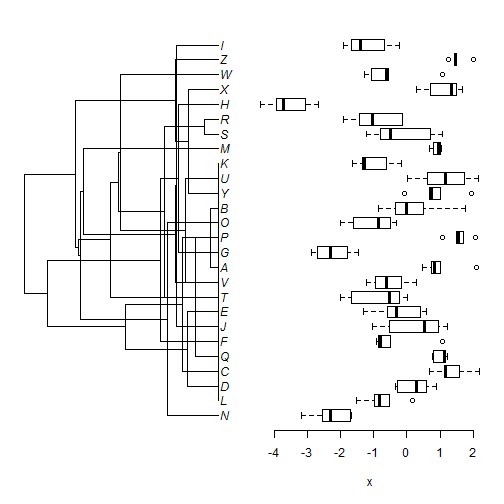This makes it much easier to see if we have a problem using the `formula` method.

The update can be seen here.

### New function to print box plot next to a tree

To complement my recent function that uses a plot array to print a bar plot next to a plotted tree (1, 2, 3), I thought I'd also show how to do the same with a plotted box plot.

Here is the function. It modifies the `plotTree.barplot` version in a couple of interesting ways:

``````plotTree.boxplot<-function(tree,x,args.plotTree=list(),
args.boxplot=list()){
cw<-reorder(tree)
if(!is.list(x)&&class(x)!="formula"){
obj<-setNames(
lapply(cw\$tip.label,function(x,y) y[which(names(y)==x)],
y=x),cw\$tip.label)
} else obj<-x
if(class(x)=="formula")
args.boxplot\$formula<-obj else args.boxplot\$x<-obj
args.boxplot\$horizontal<-TRUE
args.boxplot\$axes<-FALSE
args.boxplot\$names.arg<-""
args.boxplot\$xlim<-c(1,Ntip(cw))
if(is.null(args.boxplot\$space)) args.boxplot\$space<-0.7
if(is.null(args.boxplot\$mar))
args.boxplot\$mar<-c(5.1,0,2.1,1.1)
else args.boxplot\$mar<-0.1
args.plotTree\$tree<-cw
if(is.null(args.plotTree\$mar))
args.plotTree\$mar<-c(5.1,1.1,2.1,0)
else {
args.plotTree\$mar<-0
}
if(args.plotTree\$mar!=args.boxplot\$mar)
args.plotTree\$mar<-args.boxplot\$mar
if(args.plotTree\$mar!=args.boxplot\$mar)
args.plotTree\$mar<-args.boxplot\$mar
if(is.null(args.plotTree\$ftype)) args.plotTree\$ftype<-"i"
if(is.null(args.plotTree\$lwd)) args.plotTree\$lwd<-1
par(mfrow=c(1,2))
do.call(plotTree,args.plotTree)
par(mar=args.boxplot\$mar)
ii<-which(names(args.boxplot)%in%c("formula","x"))
args.boxplot<-c(args.boxplot[ii],args.boxplot[-ii])
obj<-do.call(boxplot,args.boxplot)
axis(1)
if(!is.null(args.boxplot\$xlab)) title(xlab=args.boxplot\$xlab)
else title(xlab="x")
invisible(obj)
}
``````

Obviously, the arguments passed to the plotting method are a little bit different - but most significantly, `boxplot` can take as its first argument either `x`, generally a list in which each element is a vector of values corresponding to the rows of the boxplot, or a formula.

Let's try it:

``````library(phytools)
## here's our tree & data
tree
``````
``````##
## Phylogenetic tree with 26 tips and 25 internal nodes.
##
## Tip labels:
##  Z, Y, X, W, V, U, ...
##
## Rooted; includes branch lengths.
``````
``````xe[1:30] ## first 30 values
``````
``````##           Z           Z           Z           Z           Z           Y
## -0.21795787 -0.32369195  0.61508766 -0.71780324 -0.09337898  0.29571288
##           Y           Y           Y           Y           X           X
##  0.63917603 -0.06377179 -0.19115456  0.20776738 -1.24725366 -0.23757952
##           X           X           X           W           W           W
## -0.99325267 -0.07823182 -0.63543671 -0.43174636 -0.95828938 -0.62417070
##           W           W           V           V           V           V
##  0.46085304  0.31871249 -1.83209464 -0.77540702 -2.05616575 -1.86725816
##           V           U           U           U           U           U
## -1.00503367 -2.28712937 -2.11539566 -1.30714430 -0.71566793 -1.00299982
``````
``````plotTree.boxplot(tree,xe) ## default
``````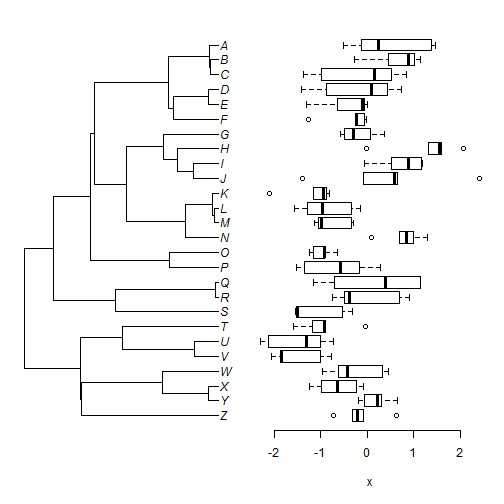``````## using formula:
spp<-factor(names(xe),
levels=reorder(tree)\$tip.label)
spp
``````
``````##    Z Z Z Z Z Y Y Y Y Y X X X X X W W W W W V V V V V U U U U U T T T T T
##   S S S S S R R R R R Q Q Q Q Q P P P P P O O O O O N N N N N M M M M M
##   L L L L L K K K K K J J J J J I I I I I H H H H H G G G G G F F F F F
##  E E E E E D D D D D C C C C C B B B B B A A A A A
## Levels: Z Y X W V U T S R Q P O N M L K J I H G F E D C B A
``````
``````plotTree.boxplot(tree,xe~spp,
args.boxplot=list(mar=c(5.1,0,4.1,1.1),
xlab="relative length (inches)"))
``````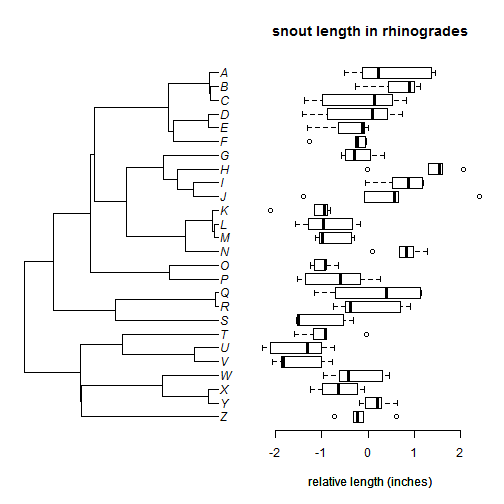One trick is that for the `formula` method we need to make sure that the levels of our factor (in this case, `spp`) are in the order of the tip labels of our cladewise re-ordered tree!

Nonetheless, hope this is a handy function for some users.

Note that this function basically encapsulates a method that I described in an earlier post, with some additional flexibility.

## Sunday, July 24, 2016

### Adding features to a phylogeny with a bar plot

I have recently posted (1, 2) on showing a bar plot next to a plotted tree. Enrico Rezende showed a figure in which the bars were colored black or white depending on whether the trait value fell below or above the trait median, respectively, and in which the median value is also overlain using a arrowhead on the abscissa combined with a vertical dashed line.

I just wanted to show (and record for posterity) how this can be duplicated, more or less, in R.

Here, I'm once again working with the eel body size data from my prior posts:

``````library(phytools)
packageVersion("phytools")
``````
``````##  '0.5.41'
``````
``````eel.tree
``````
``````##
## Phylogenetic tree with 61 tips and 60 internal nodes.
##
## Tip labels:
##  Moringua_edwardsi, Kaupichthys_nuchalis, Gorgasia_taiwanensis, Heteroconger_hassi, Venefica_proboscidea, Anguilla_rostrata, ...
##
## Rooted; includes branch lengths.
``````
``````bsize
``````
``````##                 Albula_vulpes             Anguilla_anguilla
##                         104.0                          50.0
##              Anguilla_bicolor             Anguilla_japonica
##                         120.0                         150.0
##             Anguilla_rostrata                Ariosoma_anago
##                         152.0                          60.0
##           Ariosoma_balearicum           Ariosoma_shiroanago
##                          35.0                          40.0
##        Bathyuroconger_vicinus   Brachysomophis_crocodilinus
##                          88.0                         120.0
##              Conger_japonicus              Conger_myriaster
##                         140.0                         100.0
##               Conger_verreaxi                Conger_wilsoni
##                         200.0                         150.0
##        Congresox_talabonoides            Cynoponticus_ferox
##                         250.0                         200.0
##            Dysomma_anguillare                  Elops_saurus
##                          52.0                         100.0
##         Facciolella_gilbertii          Gavialiceps_taeniola
##                          61.0                          64.7
##         Gnathophis_longicauda          Gorgasia_taiwanensis
##                          38.6                          74.1
##         Gymnothorax_castaneus   Gymnothorax_flavimarginatus
##                         150.0                         240.0
##            Gymnothorax_kidako           Gymnothorax_moringa
##                          91.5                         200.0
## Gymnothorax_pseudothyrsoideus       Gymnothorax_reticularis
##                          80.0                          60.0
##            Heteroconger_hassi          Ichthyapus_ophioneus
##                          40.0                          45.0
##      Kaupichthys_hyoproroides          Kaupichthys_nuchalis
##                          30.0                          16.0
##          Megalops_cyprinoides             Moringua_edwardsi
##                         150.0                          15.0
##             Moringua_javanica              Muraenesox_bagio
##                         120.0                         200.0
##           Muraenesox_cinereus          Myrichthys_breviceps
##                         220.0                         102.0
##          Myrichthys_maculosus         Myrichthys_magnificus
##                         100.0                          78.0
##                Myrophis_vafer        Nemichthys_scolopaceus
##                          46.0                         130.0
##          Nettastoma_melanurum        Ophichthus_serpentinus
##                          77.3                          68.0
##          Ophichthus_zophochir        Oxyconger_leptognathus
##                          88.0                          60.0
## Parabathymyrus_macrophthalmus           Paraconger_notialis
##                          47.0                          62.7
##      Pisodonophis_cancrivorus          Poeciloconger_kapala
##                         108.0                          60.0
##         Rhinomuraena_quaesita          Rhynchoconger_flavus
##                         130.0                         150.0
##        Saurenchelys_fierasfer      Scolecenchelys_breviceps
##                          50.0                          60.0
##            Scuticaria_tigrina             Serrivomer_beanii
##                         120.0                          78.0
##             Serrivomer_sector        Simenchelys_parasitica
##                          76.0                          61.0
##            Uroconger_lepturus      Uropterygius_micropterus
##                          52.0                          30.0
##          Venefica_proboscidea
##                         100.0
``````
``````col<-c("black","white")[
as.numeric(bsize[reorder(eel.tree)\$tip.label]>median(bsize))+1]
obj<-plotTree.barplot(eel.tree,bsize,list(fsize=0.6),
list(col=col,log="x",
xlim=c(10,max(bsize)),xlab="length (cm)"))
lines(rep(median(bsize),2),par()\$usr[3:4],lty="dashed",col="grey")
rw<-diff(range(bsize))
polygon(x=c(median(bsize),median(bsize)^0.98,median(bsize)^1.02),
y=c(par()\$usr,-0.5,-0.5),col="grey")
``````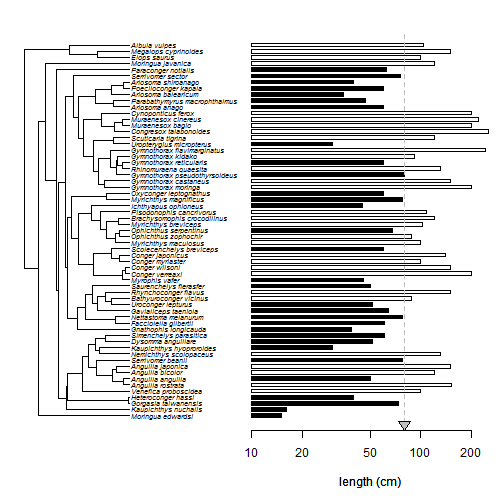The trickiest part of this, really, was figuring out how to plot the polygon on the abscissa in a way that it appeared symmetric on a log-scale!

In general, any features we would normally add to a barplot we can do it to in R. Slightly more complicated is the task of adding features to the tree. Let me think about that one.